# Random Noise Generators in Simulink

You can generate noise for communication system modeling using the MATLAB® Function block with a random number generator. This example generates and displays histogram plots of Gaussian, Rayleigh, Rician, and Uniform noise.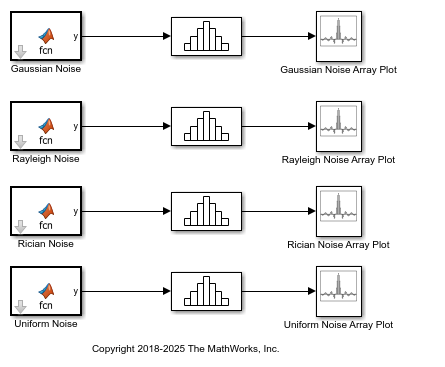The noise generators output 1e5-by-1 vectors every second, which is equivalent to a 0.00001 second sample time. In this model, each MATLAB Function block defines a specific noise generator using its underlying function. To view the underlying code for a MATLAB Function block in the MATLAB Editor, open the model, select the desired MATLAB Function block, and then press Ctrl+u. Each MATLAB function block contains block mask parameters that map to the function arguments in the underlying code.

For each MATLAB Function block the Samples per frame parameter maps to its underlying function argument `spf`. Similarly, Seed maps to `seed`.

The Gaussian Noise MATLAB Function block maps the Power (dBW) parameter to `p`, and defines the function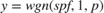The Rayleigh Noise MATLAB Function block maps the Sigma parameter to `alpha`, and defines the function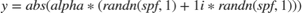The Rician Noise MATLAB Function block maps the Rician K-factor parameter to `K` and the Sigma parameter to `s`, and defines the function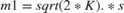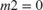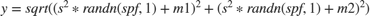The Uniform Noise MATLAB Function block maps the Noise lower bound parameter to `lb` and the Noise upper bound parameter to `ub`, and defines the function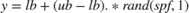The model generates these histogram plots to show the noise distribution across the spectrum for each noise generator.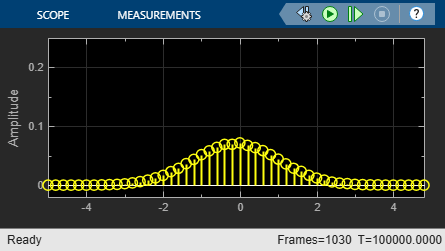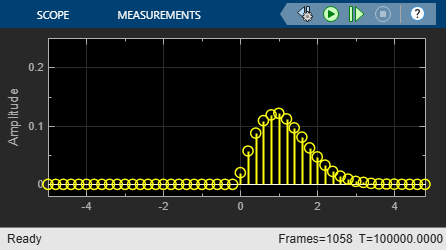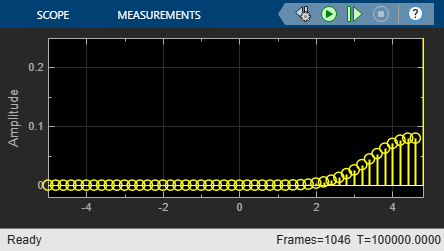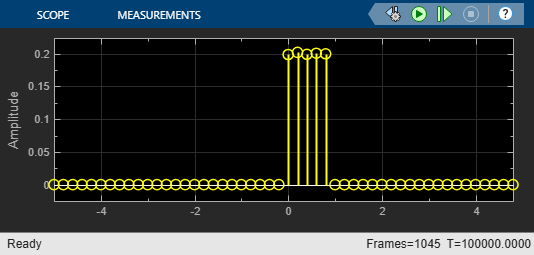For further exploration, open the model and adjust one of the noise generation settings. For example, the Rician noise generator has a K-factor of 10, which causes the mean value of the noise to be larger than that of the Rayleigh distributed noise. Double-click the Rician Noise MATLAB Function block to open the block mask and change the K-factor from 10 to 2. Rerun the model to see the noise spectrum shift.